# 1 Installations

Attach necessary libraries:

library(ASURAT)
library(SingleCellExperiment)
library(SummarizedExperiment)

# 2 Goal

A goal of ASURAT is to cluster and characterize individual samples (cells) in terms of cell type (or disease), biological function, signaling pathway activity, and so on (see here).

# 3 Quick start by SingleCellExperiment objects

Having a SingleCellExperiment object (e.g., sce), one can use ASURAT by confirming the following requirements:

• assays(sce) contains gene expression data with row and column names as variable (gene) and sample (cell), respectively,

If sce contains normalized expression data (e.g., assay(sce, "logcounts")), set assay(sce, "centered") by subtracting the data with the mean expression levels across samples (cells).

mat <- as.matrix(assay(sce, "logcounts"))
assay(sce, "centered") <- sweep(mat, 1, apply(mat, 1, mean), FUN = "-")

One may use a Seurat function Seurat::as.SingleCellExperiment() for converting Seurat objects into SingleCellExperiment ones.

Now, ready for the next step here.

# 4 Preprocessing

## 4.1 Prepare SingleCellExperiment objects

Load single-cell RNA sequencing (scRNA-seq) data.

sce <- TENxPBMCData::TENxPBMCData(dataset = c("pbmc4k"))
pbmc_counts <- as.matrix(assay(sce, "counts"))
rownames(pbmc_counts) <- make.unique(rowData(sce)$Symbol_TENx) colnames(pbmc_counts) <- make.unique(colData(sce)$Barcode)

Here, pbmc_counts is a read count table of peripheral blood mononuclear cells (PBMCs).

Below is a head of pbmc_counts:

pbmc_counts[1:5, 1:3]
#>              AAACCTGAGAAGGCCT-1 AAACCTGAGACAGACC-1 AAACCTGAGATAGTCA-1
#> RP11-34P13.3                  0                  0                  0
#> FAM138A                       0                  0                  0
#> OR4F5                         0                  0                  0
#> RP11-34P13.7                  0                  0                  0
#> RP11-34P13.8                  0                  0                  0

Create SingleCellExperiment objects by inputting gene expression data.

pbmc <- SingleCellExperiment(assays = list(counts = pbmc_counts),
rowData = data.frame(gene = rownames(pbmc_counts)),
colData = data.frame(cell = colnames(pbmc_counts)))

Check data sizes.

dim(pbmc)
#>  33694  4340

## 4.2 Control data quality

Remove variables (genes) and samples (cells) with low quality, by processing the following three steps:

1. remove variables based on expression profiles across samples,
2. remove samples based on the numbers of reads and nonzero expressed variables,
3. remove variables based on the mean read counts across samples.

First of all, add metadata for both variables and samples using ASURAT function add_metadata().

The arguments are

• sce: SingleCellExperiment object, and
• mitochondria_symbol: a string representing for mitochondrial genes.
pbmc <- add_metadata(sce = pbmc, mitochondria_symbol = "^MT-")

One can check the results in rowData(sce) and colData(sce) slots.

### 4.2.1 Remove variables based on expression profiles

ASURAT function remove_variables() removes variable (gene) data such that the numbers of non-zero expressing samples (cells) are less than min_nsamples.

pbmc <- remove_variables(sce = pbmc, min_nsamples = 10)

### 4.2.2 Remove samples based on expression profiles

Qualities of sample (cell) data are confirmed based on proper visualization of colData(sce).

df <- data.frame(x = colData(pbmc)$nReads, y = colData(pbmc)$nGenes)
ggplot2::ggplot() +
ggplot2::geom_point(ggplot2::aes(x = df$x, y = df$y), size = 1, alpha = 1) +
ggplot2::labs(x = "Number of reads", y = "Number of genes") +
ggplot2::theme_classic(base_size = 20)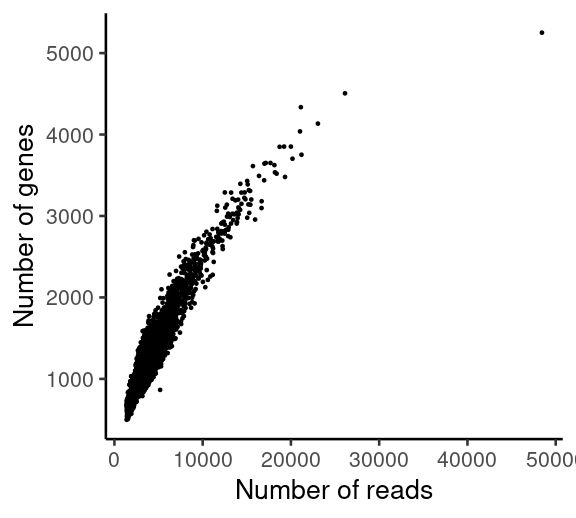df <- data.frame(x = colData(pbmc)$nReads, y = colData(pbmc)$percMT)
ggplot2::ggplot() +
ggplot2::geom_point(ggplot2::aes(x = df$x, y = df$y), size = 1, alpha = 1) +
ggplot2::labs(x = "Number of reads", y = "Perc of MT reads") +
ggplot2::theme_classic(base_size = 20)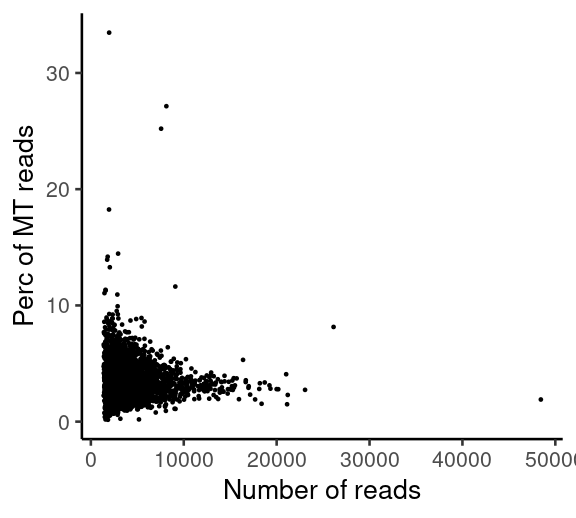ASURAT function remove_samples() removes sample (cell) data by setting cutoff values for the metadata.

The arguments are

• sce: SingleCellExperiment object,
• min_nReads and max_nReads: minimum and maximum number of reads,
• min_nGenes and max_nGenes: minimum and maximum number of non-zero expressed genes, and
• min_percMT and max_percMT: minimum and maximum percent of reads that map to mitochondrial genes, respectively. If there is no mitochondrial genes, set them as NULL.
pbmc <- remove_samples(sce = pbmc, min_nReads = 5000, max_nReads = 20000,
min_nGenes = 100, max_nGenes = 1e+10,
min_percMT = 0, max_percMT = 10)

Tips: Take care not to set excessive values for the arguments of remove_samples(), or it may produce biased results. Note that min_nReads = 5000 is somewhat large, which is used only for the tutorial.

### 4.2.3 Remove variables based on the mean read counts

Qualities of variable (gene) data are confirmed based on proper visualization of rowData(sce).

df <- data.frame(x = 1:nrow(rowData(pbmc)),
y = sort(rowData(pbmc)$nSamples, decreasing = TRUE)) ggplot2::ggplot() + ggplot2::scale_y_log10() + ggplot2::geom_point(ggplot2::aes(x = df$x, y = df$y), size = 1, alpha = 1) + ggplot2::labs(x = "Rank of genes", y = "Mean read counts") + ggplot2::theme_classic(base_size = 20)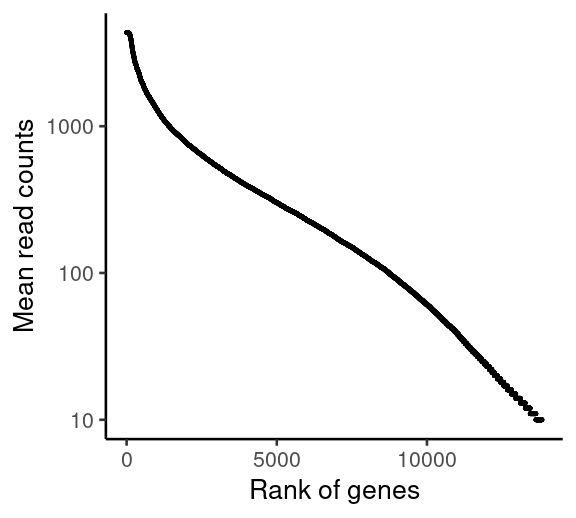ASURAT function remove_variables_second() removes variable (gene) data such that the mean read counts across samples are less than min_meannReads. pbmc <- remove_variables_second(sce = pbmc, min_meannReads = 0.05) ## 4.3 Normalize data Normalize data using log transformation with a pseudo count and center the data by subtracting with the mean expression levels across samples (cells). The resulting normalized-and-centered data are used for downstream analyses. Perform log-normalization with a pseudo count. assay(pbmc, "logcounts") <- log(assay(pbmc, "counts") + 1) Center each row data. mat <- assay(pbmc, "logcounts") assay(pbmc, "centered") <- sweep(mat, 1, apply(mat, 1, mean), FUN = "-") Set gene expression data into altExp(sce). Tips: Take care not to use a slot name “log-normalized” for altExp(sce), which may produce an error when using a Seurat (version 4.0.5) function as.Seurat() in the downstream analysis. sname <- "logcounts" altExp(pbmc, sname) <- SummarizedExperiment(list(counts = assay(pbmc, sname))) Add ENTREZ Gene IDs to rowData(sce). dictionary <- AnnotationDbi::select(org.Hs.eg.db::org.Hs.eg.db, key = rownames(pbmc), columns = "ENTREZID", keytype = "SYMBOL") dictionary <- dictionary[!duplicated(dictionary$SYMBOL), ]
rowData(pbmc)$geneID <- dictionary$ENTREZID

# 5 Multifaceted sign analysis

Infer cell or disease types, biological functions, and signaling pathway activity at the single-cell level by inputting related databases.

ASURAT transforms centered read count tables to functional feature matrices, termed sign-by-sample matrices (SSMs). Using SSMs, perform unsupervised clustering of samples (cells).

## 5.1 Compute correlation matrices

Prepare correlation matrices of gene expressions.

set.seed(1)
nrand_samples <- 1000
mat <- t(as.matrix(assay(pbmc, "centered")))
ids <- sample(rownames(mat), nrand_samples, prob = NULL)
cormat <- cor(mat[ids, ], method = "spearman")

## 5.2 Load databases

urlpath <- "https://github.com/keita-iida/ASURATDB/blob/main/genes2bioterm/"
load(url(paste0(urlpath, "20201213_human_CO.rda?raw=TRUE")))         # CO
load(url(paste0(urlpath, "20220308_human_MSigDB.rda?raw=TRUE")))     # MSigDB
load(url(paste0(urlpath, "20201213_human_GO_red.rda?raw=TRUE")))     # GO
load(url(paste0(urlpath, "20201213_human_KEGG.rda?raw=TRUE")))       # KEGG

These knowledge-based data were available from the following repositories:

Create a custom-built (CB) cell type-related database by combining different databases for analyzing human single-cell transcriptome data.

d <- list(human_CO[["cell"]], human_MSigDB[["cell"]])
res <- do.call("rbind", d)
human_CB <- list(cell = res)

Add formatted databases to metadata(sce)$sign. pbmcs <- list(CB = pbmc, GO = pbmc, KG = pbmc) metadata(pbmcs$CB) <- list(sign = human_CB[["cell"]])
metadata(pbmcs$GO) <- list(sign = human_GO[["BP"]]) metadata(pbmcs$KG) <- list(sign = human_KEGG[["pathway"]])

## 5.3 Create signs

ASURAT function remove_signs() redefines functional gene sets for the input database by removing genes, which are not included in rownames(sce), and further removes biological terms including too few or too many genes.

The arguments are

• sce: SingleCellExperiment object,
• min_ngenes: minimal number of genes> 1 (the default value is 2), and
• max_ngenes: maximal number of genes> 1 (the default value is 1000).
pbmcs$CB <- remove_signs(sce = pbmcs$CB, min_ngenes = 2, max_ngenes = 1000)
pbmcs$GO <- remove_signs(sce = pbmcs$GO, min_ngenes = 2, max_ngenes = 1000)
pbmcs$KG <- remove_signs(sce = pbmcs$KG, min_ngenes = 2, max_ngenes = 1000)

The results are stored in metadata(sce)$sign. ASURAT function cluster_genes() clusters functional gene sets using a correlation graph-based decomposition method, which produces strongly, variably, and weakly correlated gene sets (SCG, VCG, and WCG, respectively). The arguments are • sce: SingleCellExperiment object, • cormat: correlation matrix of gene expressions, • th_posi and th_nega: threshold values of positive and negative correlation coefficients, respectively. Tips: Empirically, typical values of th_posi and th_nega are $$0.15 \le {\rm th{\_}posi} \le 0.4$$ and $$-0.4 \le {\rm th{\_}nega} \le -0.15$$, but one cannot avoid trial and error for setting these values. An exhaustive parameter searching is time-consuming but helpful for obtaining interpretable results. set.seed(1) pbmcs$CB <- cluster_genesets(sce = pbmcs$CB, cormat = cormat, th_posi = 0.30, th_nega = -0.30) set.seed(1) pbmcs$GO <- cluster_genesets(sce = pbmcs$GO, cormat = cormat, th_posi = 0.30, th_nega = -0.30) set.seed(1) pbmcs$KG <- cluster_genesets(sce = pbmcs$KG, cormat = cormat, th_posi = 0.20, th_nega = -0.20) The results are stored in metadata(sce)$sign.

ASURAT function create_signs() creates signs by the following criteria:

1. the number of genes in SCG>= min_cnt_strg (the default value is 2) and
2. the number of genes in VCG>= min_cnt_vari (the default value is 2),

which are independently applied to SCGs and VCGs, respectively.

Tips: Empirically, typical values of min_cnt_strg and min_cnt_vari are $$2 \le {\rm min\_cnt\_strg} = {\rm min\_cnt\_vari} \le 4$$, but one cannot avoid trial and error for setting these values. An exhaustive parameter searching is time-consuming but helpful for obtaining interpretable results.

pbmcs$CB <- create_signs(sce = pbmcs$CB, min_cnt_strg = 2, min_cnt_vari = 2)
pbmcs$GO <- create_signs(sce = pbmcs$GO, min_cnt_strg = 4, min_cnt_vari = 4)
pbmcs$KG <- create_signs(sce = pbmcs$KG, min_cnt_strg = 3, min_cnt_vari = 3)

The results are stored in metadata(sce)$sign_all, where “CorrType” indicates SCG or VCG, “Corr” means the average correlation coefficients of SCG or VCG, “CorrWeak” means the average correlation coefficients of WCG, “CorrGene” means SCG or VCG, and “WeakCorrGene” means WCG. The orders of gene symbols and ENTREZ IDs, separated by “/”, are consistent. Tips: If one would like to recreate signs, reset the list of objects by, e.g., (pbmcs <- list(CB = pbmc, GO = pbmc, KG = pbmc)), and go back to remove_signs(). ## 5.4 Select signs If signs have semantic similarity information, one can use ASURAT function remove_signs_redundant() for removing redundant sings using the semantic similarity matrices. The arguments are • sce: SingleCellExperiment object, • similarity_matrix: a semantic similarity matrix, • threshold: a threshold value of semantic similarity, used for regarding biological terms as similar ones, and • keep_rareID: if TRUE, biological terms with the larger ICs are kept. Tips: The optimal value of threshold depends on the ontology structure as well as the method for computing semantic similarity matrix. pbmcs$GO <- remove_signs_redundant(sce = pbmcs$GO, similarity_matrix = human_GO$similarity_matrix$BP, threshold = 0.70, keep_rareID = TRUE) The results are stored in metadata(sce)$sign_SCG, metadata(sce)$sign_VCG, metadata(sce)$sign_all, and if there exist, metadata(sce)$sign_SCG_redundant and metadata(sce)$sign_VCG_redundant.

ASURAT function remove_signs_manually() removes signs by specifying IDs (e.g., GOID:XXX) or descriptions (e.g., COVID) using grepl(). The arguments are sce and keywords (keywords separated by |).

keywords <- "Covid|COVID"
pbmcs$KG <- remove_signs_manually(sce = pbmcs$KG, keywords = keywords)

The results are stored in metadata(sce)$sign_SCG, metadata(sce)$sign_VCG, and metadata(sce)$sign_all. There is another ASURAT function select_signs_manually(), a counter part of remove_signs_manually(), which removes signs that do not include keywords (keywords separated by |). keywords <- "cell|cyte" test <- select_signs_manually(sce = pbmcs$CB, keywords = keywords)

tail(assay(pbmcs$CB, "counts")[, 1:3], n = 4)) #> AAACCTGCAGGCGATA-1 AAACCTGCATGAAGTA-1 AAACCTGGTGCGGTAA-1 #> CL:0000097-S 0.2538591 0.17084896 -0.21152070 #> CL:0000100-S 0.4204088 0.30813515 -0.30692790 #> CL:0000121-S 0.1317555 0.42244834 -0.02095966 #> CL:0000127-S 0.1319279 0.43485496 -0.23608112 #> MSigDBID:265-V 0.2879127 0.01622889 -0.05623750 #> MSigDBID:266-V 0.2971490 0.07279125 -0.18265357 #> MSigDBID:276-V 0.3259615 -0.06231676 0.03452386 #> MSigDBID:293-V 0.1557055 0.12024693 -0.12444035 ## 5.6 Reduce dimensions of sign-by-sample matrices Perform principal component analysis and t-distributed stochastic neighbor embedding (t-SNE). pca_dims <- c(30, 30, 50) tsne_dims <- c(2, 2, 3) for(i in seq_along(pbmcs)){ set.seed(1) mat <- t(as.matrix(assay(pbmcs[[i]], "counts"))) res <- Rtsne::Rtsne(mat, dim = tsne_dims[i], pca = TRUE, initial_dims = pca_dims[i]) reducedDim(pbmcs[[i]], "TSNE") <- res[["Y"]] } Show the results of dimensional reduction in t-SNE spaces. df <- as.data.frame(reducedDim(pbmcs$CB, "TSNE"))
ggplot2::ggplot() + ggplot2::geom_point(ggplot2::aes(x = df[, 1], y = df[, 2]),
color = "black", size = 1, alpha = 1) +
ggplot2::labs(title = "PBMC (cell type)", x = "tSNE_1", y = "tSNE_2") +
ggplot2::theme_classic(base_size = 15)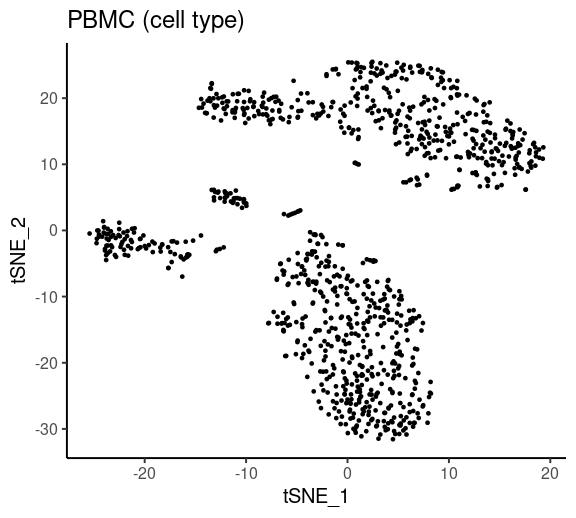df <- as.data.frame(reducedDim(pbmcs$GO, "TSNE")) ggplot2::ggplot() + ggplot2::geom_point(ggplot2::aes(x = df[, 1], y = df[, 2]), color = "black", size = 1, alpha = 1) + ggplot2::labs(title = "PBMC (function)", x = "tSNE_1", y = "tSNE_2") + ggplot2::theme_classic(base_size = 15)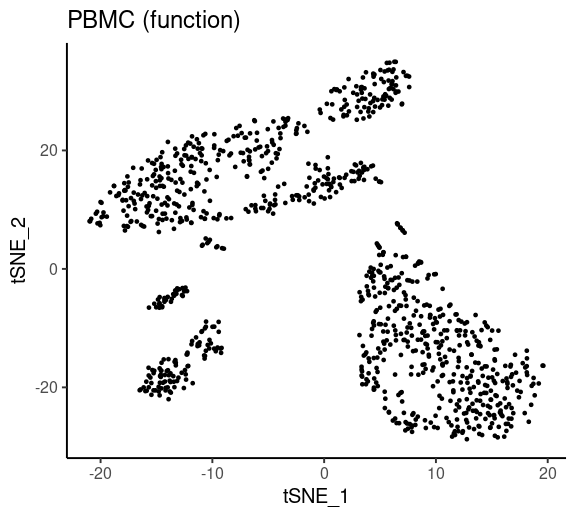Use ASURAT function plot_dataframe3D() for plotting three-dimensional data. See ?plot_dataframe3D for details. df <- as.data.frame(reducedDim(pbmcs$KG, "TSNE"))
plot_dataframe3D(dataframe3D = df, theta = 45, phi = 30, title = "PBMC (pathway)",
xlabel = "tSNE_1", ylabel = "tSNE_2", zlabel = "tSNE_3")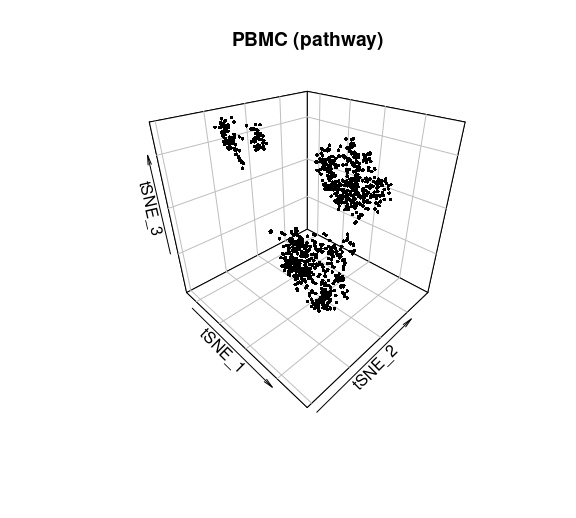## 5.7 Cluster cells

### 5.7.1 Use Seurat functions

To date (December, 2021), one of the most useful clustering methods in scRNA-seq data analysis is a combination of a community detection algorithm and graph-based unsupervised clustering, developed in Seurat package.

Here, our strategy is as follows:

1. convert SingleCellExperiment objects into Seurat objects (note that rowData() and colData() must have data),
2. perform ScaleData(), RunPCA(), FindNeighbors(), and FindClusters(),
3. convert Seurat objects into temporal SingleCellExperiment objects temp,
4. add colData(temp)$seurat_clusters into colData(sce)$seurat_clusters.
resolutions <- c(0.20, 0.20, 0.10)
ds <- list(seq_len(20), seq_len(20), seq_len(20))
for(i in seq_along(pbmcs)){
surt <- Seurat::as.Seurat(pbmcs[[i]], counts = "counts", data = "counts")
mat <- as.matrix(assay(pbmcs[[i]], "counts"))
surt[["SSM"]] <- Seurat::CreateAssayObject(counts = mat)
Seurat::DefaultAssay(surt) <- "SSM"
surt <- Seurat::ScaleData(surt, features = rownames(surt))
surt <- Seurat::RunPCA(surt, features = rownames(surt))
surt <- Seurat::FindNeighbors(surt, reduction = "pca", dims = ds[[i]])
surt <- Seurat::FindClusters(surt, resolution = resolutions[i])
temp <- Seurat::as.SingleCellExperiment(surt)
colData(pbmcs[[i]])$seurat_clusters <- colData(temp)$seurat_clusters
}

Show the clustering results in t-SNE spaces.

labels <- colData(pbmcs$CB)$seurat_clusters
df <- as.data.frame(reducedDim(pbmcs$CB, "TSNE")) ggplot2::ggplot() + ggplot2::geom_point(ggplot2::aes(x = df[, 1], y = df[, 2], color = labels), size = 1, alpha = 1) + ggplot2::labs(title = "PBMC (cell type)", x = "tSNE_1", y = "tSNE_2", color = "") + ggplot2::theme_classic(base_size = 15) + ggplot2::scale_colour_hue() + ggplot2::guides(colour = ggplot2::guide_legend(override.aes = list(size = 4)))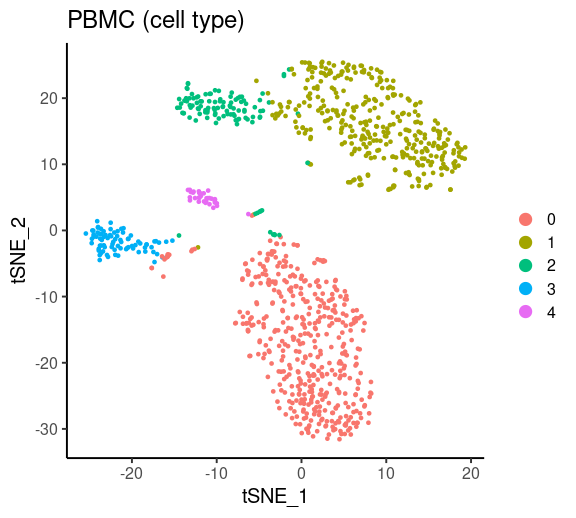labels <- colData(pbmcs$GO)$seurat_clusters df <- as.data.frame(reducedDim(pbmcs$GO, "TSNE"))
ggplot2::ggplot() +
ggplot2::geom_point(ggplot2::aes(x = df[, 1], y = df[, 2], color = labels),
size = 1, alpha = 1) +
ggplot2::labs(title = "PBMC (function)", x = "tSNE_1", y = "tSNE_2", color = "") +
ggplot2::theme_classic(base_size = 15) +
ggplot2::scale_colour_brewer(palette = "Set1") +
ggplot2::guides(colour = ggplot2::guide_legend(override.aes = list(size = 4)))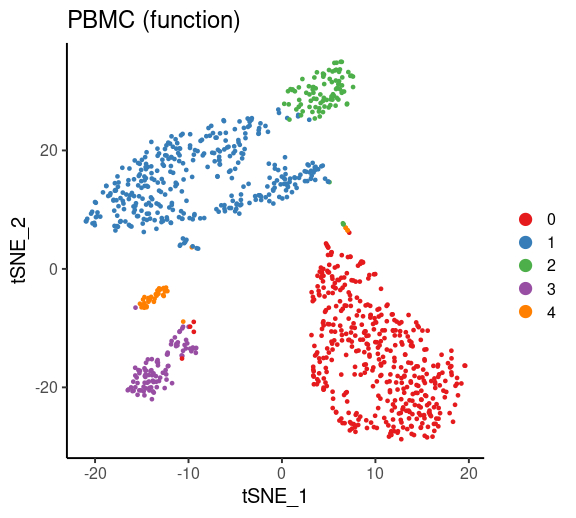Use ASURAT function plot_dataframe3D() for plotting three-dimensional data. See ?plot_dataframe3D for details.

labels <- colData(pbmcs$KG)$seurat_clusters
colors <- scales::brewer_pal(palette = "Set2")(length(unique(labels)))[labels]
df <- as.data.frame(reducedDim(pbmcs$KG, "TSNE")[, seq_len(3)]) plot_dataframe3D(dataframe3D = df, labels = labels, colors = colors, theta = 45, phi = 30, title = "PBMC (pathway)", xlabel = "tSNE_1", ylabel = "tSNE_2", zlabel = "tSNE_3")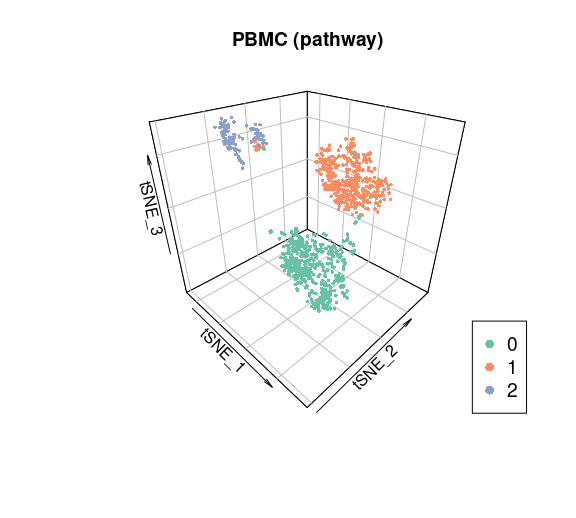### 5.7.2 Cell cycle inference using Seurat functions If there is gene expression data in altExp(sce), one can infer cell cycle phases by using Seurat functions in the similar manner as above. surt <- Seurat::as.Seurat(pbmcs$CB, counts = "counts", data = "counts")
mat <- as.matrix(assay(altExp(pbmcs$CB), "counts")) surt[["GEM"]] <- Seurat::CreateAssayObject(counts = mat) Seurat::DefaultAssay(surt) <- "GEM" surt <- Seurat::ScaleData(surt, features = rownames(surt)) surt <- Seurat::RunPCA(surt, features = rownames(surt)) surt <- Seurat::CellCycleScoring(surt, s.features = Seurat::cc.genes$s.genes,
g2m.features = Seurat::cc.genes$g2m.genes) temp <- Seurat::as.SingleCellExperiment(surt) colData(pbmcs$CB)$Phase <- colData(temp)$Phase

## 5.8 Investigate significant signs

Significant signs are analogous to differentially expressed genes but bear biological meanings. Note that naïve usages of statistical tests should be avoided because the row vectors of SSMs are centered.

Instead, ASURAT function compute_sepI_all() computes separation indices for each cluster against the others. Briefly, a separation index “sepI”, ranging from -1 to 1, is a nonparametric measure of significance of a given sign score for a given subpopulation. The larger (resp. smaller) sepI is, the more reliable the sign is as a positive (resp. negative) marker for the cluster.

The arguments are

• sce: SingleCellExperiment object,
• labels: a vector of labels of all the samples, and
• nrand_samples: an integer for the number of samples used for random sampling, which samples at least one sample per cluster.
for(i in seq_along(pbmcs)){
set.seed(1)
labels <- colData(pbmcs[[i]])$seurat_clusters pbmcs[[i]] <- compute_sepI_all(sce = pbmcs[[i]], labels = labels, nrand_samples = 200) } The results are stored in metadata(sce)$marker_signs.

When computing separation indices between given clusters, e.g., cluster 1 versus clusters 2 and 3, use an ASURAT function compute_sepI_clusters(). See ?compute_sepI_clusters for details.

## 5.9 Investigate significant genes

### 5.9.1 Use Seurat function

To date (December, 2021), one of the most useful methods of multiple statistical tests in scRNA-seq data analysis is to use a Seurat function FindAllMarkers().

If there is gene expression data in altExp(sce), one can investigate differentially expressed genes by using Seurat functions in the similar manner as described before.

set.seed(1)
surt <- Seurat::as.Seurat(pbmcs$CB, counts = "counts", data = "counts") mat <- as.matrix(assay(altExp(pbmcs$CB), "counts"))
surt[["GEM"]] <- Seurat::CreateAssayObject(counts = mat)
Seurat::DefaultAssay(surt) <- "GEM"
surt <- Seurat::SetIdent(surt, value = "seurat_clusters")
res <- Seurat::FindAllMarkers(surt, only.pos = TRUE,
min.pct = 0.50, logfc.threshold = 0.50)
metadata(pbmcs$CB)$marker_genes$all <- res ## 5.10 Multifaceted analysis Simultaneously analyze multiple sign-by-sample matrices, which helps us characterize individual samples (cells) from multiple biological aspects. ASURAT function plot_multiheatmaps() shows heatmaps (ComplexHeatmap object) of sign scores and gene expression levels (if there are), where rows and columns stand for sign (or gene) and sample (cell), respectively. See ?plot_multiheatmaps for details. First, remove unrelated signs by setting keywords, followed by selecting top significant signs and genes for the clustering results with respect to separation index and adjusted p-value, respectively. # Significant signs marker_signs <- list() keywords <- "MESENCHYMAL|LIMB|PANCREAS" for(i in seq_along(pbmcs)){ marker_signs[[i]] <- metadata(pbmcs[[i]])$marker_signs$all marker_signs[[i]] <- marker_signs[[i]][!grepl(keywords, marker_signs[[i]]$Description), ]
marker_signs[[i]] <- dplyr::group_by(marker_signs[[i]], Ident_1)
marker_signs[[i]] <- dplyr::slice_max(marker_signs[[i]], sepI, n = 2)
marker_signs[[i]] <- dplyr::slice_min(marker_signs[[i]], Rank, n = 1)
}
# Significant genes
marker_genes_CB <- metadata(pbmcs$CB)$marker_genes$all marker_genes_CB <- dplyr::group_by(marker_genes_CB, cluster) marker_genes_CB <- dplyr::slice_min(marker_genes_CB, p_val_adj, n = 2) marker_genes_CB <- dplyr::slice_max(marker_genes_CB, avg_log2FC, n = 1) Next, prepare the arguments. # ssm_list sces_sub <- list() ; ssm_list <- list() for(i in seq_along(pbmcs)){ sces_sub[[i]] <- pbmcs[[i]][rownames(pbmcs[[i]]) %in% marker_signs[[i]]$SignID, ]
ssm_list[[i]] <- assay(sces_sub[[i]], "counts")
}
names(ssm_list) <- c("SSM_cell", "SSM_function", "SSM_pathway")
# gem_list
expr_sub <- altExp(pbmcs$CB, "logcounts") expr_sub <- expr_sub[rownames(expr_sub) %in% marker_genes_CB$gene]
gem_list <- list(GeneExpr = assay(expr_sub, "counts"))
# ssmlabel_list
labels <- list() ; ssmlabel_list <- list()
for(i in seq_along(pbmcs)){
tmp <- colData(sces_sub[[i]])$seurat_clusters labels[[i]] <- data.frame(label = tmp) n_groups <- length(unique(tmp)) if(i == 1){ labels[[i]]$color <- scales::hue_pal()(n_groups)[tmp]
}else if(i == 2){
labels[[i]]$color <- scales::brewer_pal(palette = "Set1")(n_groups)[tmp] }else if(i == 3){ labels[[i]]$color <- scales::brewer_pal(palette = "Set2")(n_groups)[tmp]
}
ssmlabel_list[[i]] <- labels[[i]]
}
names(ssmlabel_list) <- c("Label_cell", "Label_function", "Label_pathway")
# gemlabel_list
label_CC <- data.frame(label = colData(pbmcs$CB)$Phase, color = NA)
gemlabel_list <- list(CellCycle = label_CC)

Show heatmaps for the selected signs and genes.

set.seed(1)
plot_multiheatmaps(ssm_list = ssm_list, gem_list = gem_list,
ssmlabel_list = ssmlabel_list, gemlabel_list = gemlabel_list,
nrand_samples = 100, show_row_names = TRUE, title = "PBMC")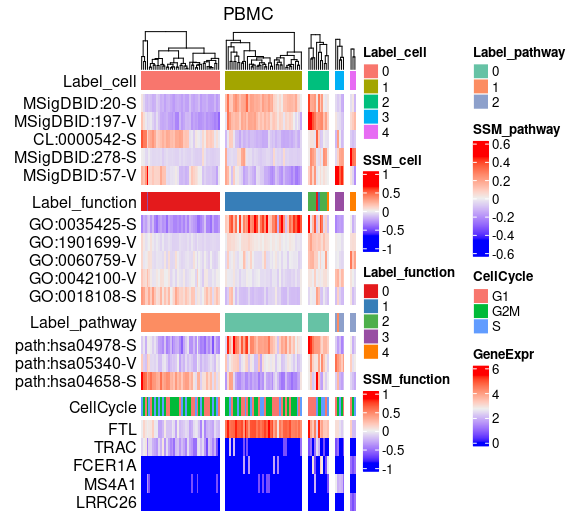Show violin plots for sign score distributions across cell type-related clusters.

labels <- colData(pbmcs$CB)$seurat_clusters
variable <- "GO:0042100-V"
description <- "B cell proliferation"
subsce <- pbmcs$GO[which(rownames(pbmcs$GO) == variable), ]
df <- as.data.frame(t(as.matrix(assay(subsce, "counts"))))
ggplot2::ggplot() +
ggplot2::geom_violin(ggplot2::aes(x = as.factor(labels), y = df[, 1],
fill = labels), trim = FALSE, size = 0.5) +
ggplot2::geom_boxplot(ggplot2::aes(x = as.factor(labels), y = df[, 1]),
width = 0.15, alpha = 0.6) +
ggplot2::labs(title = paste0(variable, "\n", description),
x = "Cluster (cell type)", y = "Sign score") +
ggplot2::theme_classic(base_size = 20) +
ggplot2::theme(legend.position = "none") + ggplot2::scale_fill_hue()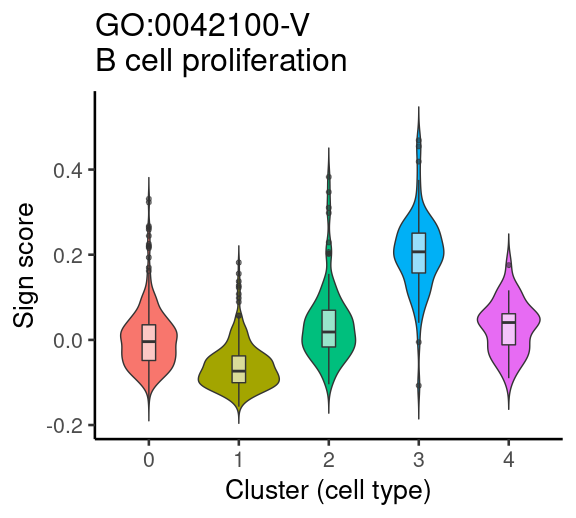Show violin plots for gene expression distributions across cell type-related clusters.

vname <- "CD79A"
sub <- altExp(pbmcs$CB, "logcounts") sub <- sub[rownames(sub) == vname, ] labels <- colData(pbmcs$CB)\$seurat_clusters
df <- as.data.frame(t(assay(sub, "counts")))
ggplot2::ggplot() +
ggplot2::geom_violin(ggplot2::aes(x = as.factor(labels), y = df[, 1],
fill = labels), trim = FALSE, size = 0.5) +
ggplot2::geom_boxplot(ggplot2::aes(x = as.factor(labels), y = df[, 1]),
width = 0.15, alpha = 0.6) +
ggplot2::labs(title = vname, x = "Cluster (cell type)",
y = "Normalized expression") +
ggplot2::theme_classic(base_size = 20) +
ggplot2::theme(legend.position = "none") + ggplot2::scale_fill_hue()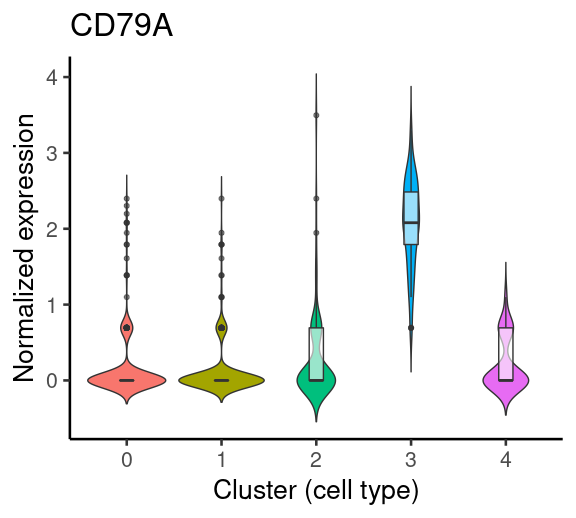# 6 Session information

sessionInfo()
#> R version 4.2.0 RC (2022-04-19 r82224)
#> Platform: x86_64-pc-linux-gnu (64-bit)
#> Running under: Ubuntu 20.04.4 LTS
#>
#> Matrix products: default
#> BLAS:   /home/biocbuild/bbs-3.15-bioc/R/lib/libRblas.so
#> LAPACK: /home/biocbuild/bbs-3.15-bioc/R/lib/libRlapack.so
#>
#> locale:
#>   LC_CTYPE=en_US.UTF-8       LC_NUMERIC=C
#>   LC_TIME=en_GB              LC_COLLATE=C
#>   LC_MONETARY=en_US.UTF-8    LC_MESSAGES=en_US.UTF-8
#>   LC_PAPER=en_US.UTF-8       LC_NAME=C
#>   LC_ADDRESS=C               LC_TELEPHONE=C
#>  LC_MEASUREMENT=en_US.UTF-8 LC_IDENTIFICATION=C
#>
#> attached base packages:
#>  stats4    stats     graphics  grDevices utils     datasets  methods
#>  base
#>
#> other attached packages:
#>   TENxPBMCData_1.13.0         HDF5Array_1.24.0
#>   rhdf5_2.40.0                DelayedArray_0.22.0
#>   Matrix_1.4-1                SingleCellExperiment_1.18.0
#>   SummarizedExperiment_1.26.0 Biobase_2.56.0
#>   GenomicRanges_1.48.0        GenomeInfoDb_1.32.0
#>  IRanges_2.30.0              S4Vectors_0.34.0
#>  BiocGenerics_0.42.0         MatrixGenerics_1.8.0
#>  matrixStats_0.62.0          ASURAT_1.0.0
#>
#> loaded via a namespace (and not attached):
#>    utf8_1.2.2                    reticulate_1.24
#>    tidyselect_1.1.2              RSQLite_2.2.12
#>    AnnotationDbi_1.58.0          htmlwidgets_1.5.4
#>    grid_4.2.0                    Rtsne_0.16
#>    munsell_0.5.0                 codetools_0.2-18
#>   ica_1.0-2                     future_1.25.0
#>   miniUI_0.1.1.1                misc3d_0.9-1
#>   withr_2.5.0                   spatstat.random_2.2-0
#>   colorspace_2.0-3              filelock_1.0.2
#>   highr_0.9                     knitr_1.38
#>   Seurat_4.1.0                  ROCR_1.0-11
#>   tensor_1.5                    listenv_0.8.0
#>   labeling_0.4.2                GenomeInfoDbData_1.2.8
#>   polyclip_1.10-0               bit64_4.0.5
#>   farver_2.1.0                  parallelly_1.31.1
#>   vctrs_0.4.1                   generics_0.1.2
#>   xfun_0.30                     BiocFileCache_2.4.0
#>   R6_2.5.1                      doParallel_1.0.17
#>   clue_0.3-60                   bitops_1.0-7
#>   rhdf5filters_1.8.0            spatstat.utils_2.3-0
#>   cachem_1.0.6                  assertthat_0.2.1
#>   promises_1.2.0.1              scales_1.2.0
#>   gtable_0.3.0                  Cairo_1.5-15
#>   globals_0.14.0                goftest_1.2-3
#>   rlang_1.0.2                   GlobalOptions_0.1.2
#>   splines_4.2.0                 lazyeval_0.2.2
#>   spatstat.geom_2.4-0           BiocManager_1.30.17
#>   yaml_2.3.5                    reshape2_1.4.4
#>   abind_1.4-5                   httpuv_1.6.5
#>   tools_4.2.0                   tcltk_4.2.0
#>   ggplot2_3.3.5                 ellipsis_0.3.2
#>   spatstat.core_2.4-2           jquerylib_0.1.4
#>   RColorBrewer_1.1-3            ggridges_0.5.3
#>   Rcpp_1.0.8.3                  plyr_1.8.7
#>   zlibbioc_1.42.0               purrr_0.3.4
#>   RCurl_1.98-1.6                rpart_4.1.16
#>   deldir_1.0-6                  pbapply_1.5-0
#>   GetoptLong_1.0.5              cowplot_1.1.1
#>   zoo_1.8-10                    SeuratObject_4.0.4
#>   ggrepel_0.9.1                 cluster_2.1.3
#>   magrittr_2.0.3                magick_2.7.3
#>   data.table_1.14.2             scattermore_0.8
#>   circlize_0.4.14               lmtest_0.9-40
#>   RANN_2.6.1                    fitdistrplus_1.1-8
#>   patchwork_1.1.1               mime_0.12
#>   evaluate_0.15                 xtable_1.8-4
#>   gridExtra_2.3                 shape_1.4.6
#>   compiler_4.2.0                tibble_3.1.6
#>   KernSmooth_2.23-20            crayon_1.5.1
#>   htmltools_0.5.2               mgcv_1.8-40
#>  later_1.3.0                   tidyr_1.2.0
#>  DBI_1.1.2                     ExperimentHub_2.4.0
#>  dbplyr_2.1.1                  ComplexHeatmap_2.12.0
#>  MASS_7.3-57                   rappdirs_0.3.3
#>  cli_3.3.0                     parallel_4.2.0
#>  igraph_1.3.1                  pkgconfig_2.0.3
#>  plotly_4.10.0                 spatstat.sparse_2.1-1
#>  foreach_1.5.2                 bslib_0.3.1
#>  XVector_0.36.0                stringr_1.4.0
#>  digest_0.6.29                 sctransform_0.3.3
#>  RcppAnnoy_0.0.19              spatstat.data_2.2-0
#>  Biostrings_2.64.0             rmarkdown_2.14
#>  leiden_0.3.9                  uwot_0.1.11
#>  curl_4.3.2                    shiny_1.7.1
#>  rjson_0.2.21                  lifecycle_1.0.1
#>  nlme_3.1-157                  jsonlite_1.8.0
#>  Rhdf5lib_1.18.0               limma_3.52.0
#>  viridisLite_0.4.0             fansi_1.0.3
#>  pillar_1.7.0                  lattice_0.20-45
#>  KEGGREST_1.36.0               fastmap_1.1.0
#>  httr_1.4.2                    survival_3.3-1
#>  interactiveDisplayBase_1.34.0 glue_1.6.2
#>  png_0.1-7                     iterators_1.0.14
#>  plot3D_1.4                    BiocVersion_3.15.2
#>  bit_4.0.4                     stringi_1.7.6
#>  sass_0.4.1                    blob_1.2.3
#>  org.Hs.eg.db_3.15.0           AnnotationHub_3.4.0
#>  memoise_2.0.1                 dplyr_1.0.8
#>  irlba_2.3.5                   future.apply_1.9.0